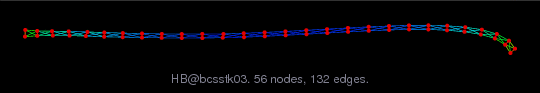Matrix: HB/bcsstk03

Description: SYMMETRIC STIFFNESS MATRIX, SMALL TEST STRUCTURE(undirected graph drawing)• Matrix group: HB
• download as a MATLAB mat-file, file size: 3 KB. Use UFget(25) or UFget('HB/bcsstk03') in MATLAB.

 Matrix properties number of rows 112 number of columns 112 nonzeros 640 structural full rank? yes structural rank 112 # of blocks from dmperm 2 # strongly connected comp. 2 explicit zero entries 0 nonzero pattern symmetry symmetric numeric value symmetry symmetric type real structure symmetric Cholesky candidate? yes positive definite? yes

 author J. Lewis editor I. Duff, R. Grimes, J. Lewis date 1982 kind structural problem 2D/3D problem? yes

 Ordering statistics: result nnz(chol(P*(A+A'+s*I)*P')) with AMD 384 Cholesky flop count 1.4e+03 nnz(L+U), no partial pivoting, with AMD 656 nnz(V) for QR, upper bound nnz(L) for LU, with COLAMD 388 nnz(R) for QR, upper bound nnz(U) for LU, with COLAMD 596

 SVD-based statistics: norm(A) 1.99734e+11 min(svd(A)) 29410.2 cond(A) 6.79133e+06 rank(A) 112 sprank(A)-rank(A) 0 null space dimension 0 full numerical rank? yes

 singular values (MAT file): click here SVD method used: s = svd (full (A)) ; status: ok